## Example Questions

← Previous 1

### Example Question #12 : Venn Diagrams

100 students are in the 10th grade class. 30 are swimmers, 40 are runners, and 20 are swimmers and runners. What is the probability that a student is a swimmer OR a runner?

2/3

1/3

1/2

1/5

1/4

1/2

Explanation:

The formula for intersection is P(a or b) = P(a) + P(b) – P(a and b).

Now, 30 students out of 100 swim, so P(swim) = 30/100 = 3/10.

40 students run out of 100, so P(run) = 40/100 = 4/10. Notice how we are keeping 10 as the common denominator even though we could simplify this further. Keeping all of the fractions similar will make the addition and subtraction easier later on.

Finally, 20 students swim AND run, so P(swim AND run) = 20/100 = 2/10. (Again, we keep this as 2/10 instead of 1/5 so that we can combine the three fractions more easily.)

P(swim OR run) = P(swim) + P(run) – P(swim and run)

= 3/10 + 4/10 – 2/10 = 5/10 = 1/2.

### Example Question #1 : How To Find The Intersection Of A Venn Diagram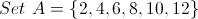and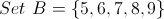Find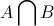.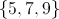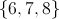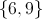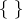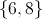Explanation:

The intersection of two sets contains every element that is present in both sets, sois the correct answer.

### Example Question #2 : How To Find The Intersection Of A Venn Diagram

We have two sports clubs offered to a class of 100 students. 70 students joined the basketball club, 40 students joined the swimming club, and 10 students joined neither. How many students joined both the swimming club and the basketball club?Explanation:

The idea is to draw a Venn Diagram and find the intersection. We have one circle of 70 and another with 40. When we add the two circles plus the 10 students who joined neither, we should get 100 students. However, when adding the two circles, we are adding the intersections twice, therefore we need to subtract the intersection once.

We get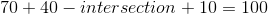, which means the intersection is 20.

### Example Question #15 : Venn Diagrams

High school freshmen can take Biology, Chemistry, or both. Iffreshmen take Biology,freshmen take Chemistry, and there arefreshmen in total. How many freshmen take both Biology and Chemistry?Explanation:

If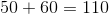students are enrolled in sciences, but there are onlystudents then we must find how many overlap in the subjects they take.

To do this we can subtractfrom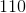.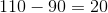Therefore,of those "enrollments" must be doubles.

Thosestudents take both Chemistry and Biology.

### Example Question #1 : How To Find The Intersection Of A Venn Diagram

Fifty 6th graders were asked what their favorite school subjects were.  Three students like math, science and English.  Five students liked math and science.  Seven students liked math and English.  Eight people liked science and English.  Twenty students liked science.  Twenty-eight students liked English.  Fourteen students liked math.  How many students didn’t like any of these classes?

3

5

10

7

None of the answers are correct

5

Explanation:

Draw a Venn diagram with three subsets:  Math, Science, and English.  Start in the center with students that like all three subjects.  Next, look at students that liked two subjects.  Be sure to subtract out the ones already counted in the middle.  Then, look at the students that only like one subject.  Be sure to subtract out the students already accounted for.  Once all of the subsets are filled, look at those students who don’t like any of these subjects.  To find the students who don’t like any of these subjects add all of the students who like at least one subject from the total number of students surveyed, which is 50.

M = math

S = science

E = English

M∩S∩E = 3

M∩S = 5 (but 3 are already accounted for) so 2 for M and S ONLY

M∩E = 7 (but 3 are already accounted for) so 4 for M and E ONLY

S∩E = 8 (but 3 are already accounted for) so 5 for S and E ONLY

M = 14 (but 3 + 2 + 4 are already accounted for) so 5 for M ONLY

S = 20 (but 3 + 2 + 5 are already accounted for) so 10 for S ONLY

E = 28 (but 3 + 4 + 5 are already accounted for) so 16 for E ONLY

Therefore, the students already accounted for is 3 + 2 +4 + 5 + 5 + 10 + 16 = 45 students

So, those students who don’t like any of these subjects are 50 – 45 = 5 students

### Example Question #1 : Venn Diagrams

Set A contains the positive even integers less than 14. Set B contains the positive multiples of three less than 20. What is the intersection of the two sets?

A∩B = {  }

A∩B = {6, 12}

A∩B = {4, 6, 8}

A∩B = {6}

A∩B = {6, 12, 18}

A∩B = {6, 12}

Explanation:

A = {2, 4, 6, 8, 10, 12}

B = {3, 6, 9, 12, 15, 18}

The intersection of a set means that the elements are in both sets: A∩B = {6, 12}

### Example Question #1 : How To Find The Intersection Of A Venn Diagram

Students at a local high school are given the option to take one gym class, one music class or one of each. Out of 100 students, 60 say that they are currently taking a gym class and 70 say that they are taking a music class. How many students are taking both?Explanation:

This problem can be solved two ways, with a formula or with reason.

Using the formula, the intersection of the Venn diagram for which classes students take is: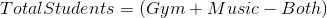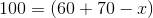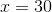By using reason, it is clear that 60 + 70 is greater than 100 by 30. It is assumed that this extra 30 students come from students who were counted twice because they took both classes.

### Example Question #1 : How To Find The Intersection Of A Venn Diagram

At a certain college, some members of the baseball team are seniors and all seniors are in statistics class.  Which statement is must be true?

Statistics class is required for the baseball team.

At least some of the baseball team are taking statistics.

No member of the baseball team takes statistics.

All members of the baseball team take statistics.

None of these can be determined.

At least some of the baseball team are taking statistics.

Explanation:

The statement says all seniors take statistics so if you are a senior you are in statistics automatically.  It also said some baseball team members are seniors which means at least some teammates must be in statistics.

### Example Question #2 : How To Find The Intersection Of A Venn Diagram

Let Set A =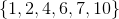and Set B =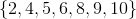.

Find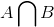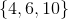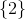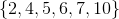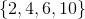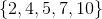Explanation:represents the intersection of the two sets. In other words, we want all the elements that appear in both sets. The elements that appear in both sets are 2, 4, 6, and 10.

Therefore,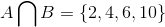### Example Question #1 : How To Find The Intersection Of A Venn Diagram

There are  75 juniors at a high school. 15 of the students are enrolled in Physics and 40 students are enrolled in Chemistry. 30 students are not enrolled in either Physics or Chemistry. How many students are enrolled in both Physics and Chemistry?

5

30

25

15

10

10

Explanation:

First, subtract the students that are in neither class; 75 – 30 = 45 students.

Thus, 45 students are enrolled in Chemistry, Physics, or both.  Of these 45 students, we know 40 are in Chemistry, so that leaves 5 students who are enrolled in Physics only; with 15 total students in Physics, that means 10 must be in Chemistry as well. So 10 students are in both Physics and Chemistry.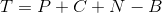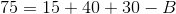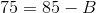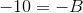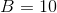← Previous 1